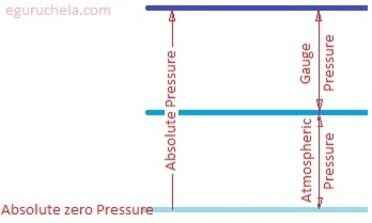### Absolute Pressure Calculation

The calculator can calculate the absolute pressure or gauge pressure or atmospheric pressure as selected from drop-down based on given appropriate values. Pressure is the force per unit area applied to any object in a direction perpendicular to the surface. Pressure is defined as the normal force per unit area of a surface.
Absolute Pressure: Pa = Pg + Pat
Gauge Pressure: Pg = Pa - Pat
Atmospheric Pressure: Pat = Pa - Pg
where, Pa = Absolute Pressure, Pg = Gauge Pressure, Pat = Atmospheric Pressure.I want to calculate Absolute Pressure(Pa) Gauge Pressure(Pg) Atmospheric Pressure(Pat) Gauge Pressure(Pg) = P Atmospheric Pressure(Pat) = P Absolute Pressure(Pa) = P
 Pressure Type Definition Formula Absolute Pressure (Pa) The absolute pressure is the pressure of having no matter inside a space or perfect vacuum. The measurement of barometric pressure is the example of an absolute referenced pressure. Pa = Pg + Pat Gauge Pressure (Pg) The gauge pressure is measured in relation to ambient atmospheric pressure. The changes in atmospheric pressure is due to weather conditions. The gauge pressure higher than ambient pressure is referred to as positive pressure and the gauge pressure is lower than atmospheric pressure referred to as negative or vacuum gauge pressure. Pg = Pa - Pat Atmospheric Pressure (Pat) Because of unequal solar heating over the earth by sun, the temperatures varies over the entire globe. Air at the equator is much warmer than at poles. Therefore heavier air sinks toward the equator and warm (light) air rises and get spread towards the pole. The atmospheric pressure relate to daily weather patterns. The barometer is used to measure atmospheric pressure. Pat = Pa - Pg